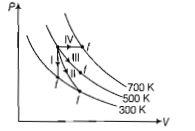Thermodynamic process are indicated in the following diagramMatch the following:

column-I            column-II

Q.       Process-II      (b)   Isobaric

R.       Process-III     (c)   Isochoric

S.       Process-IV     (d)   Isothermal

(a) $P\to a,Q\to c,R\to d,S\to b$

(b)$P\to c,Q\to a,R\to d,S\to b$

(c)$P\to c,Q\to d,R\to b,S\to a$

(d)$P\to c,Q\to d,R\to b,S\to a$

Concept Videos :-

#3 | Thermodynamic Processes
#4 | Isobaric Process
#5 | Isochoric & Isothermal Process
#6 | Interconversion of Graphs
#7 | Solved Example: 1
#8 | Solved Example: 2